# Three piles

100 kg of sugar we divide into three piles. The first pile is small. If we added to second 2 kg of sugar it would have 25% more sugar than the first pile. If we added to third pile 3 kg of sugar it would have 20% more sugar than the 2nd pile. How many kilograms of sugar are on each pile?

Result

a =  28.64
b =  33.8
c =  37.56

#### Solution:

a+b+c=100
b+2 = 1.25 a
c+3 = 1.20 b

a+b+c = 100
1.25a-b = 2
1.2b-c = 3

a = 71625 = 28.64
b = 1695 = 33.8
c = 93925 = 37.56

Calculated by our linear equations calculator.

Leave us a comment of example and its solution (i.e. if it is still somewhat unclear...):

Showing 0 comments:Be the first to comment!#### To solve this example are needed these knowledge from mathematics:

Our percentage calculator will help you quickly calculate various typical tasks with percentages. Do you have a linear equation or system of equations and looking for its solution? Or do you have quadratic equation?

## Next similar examples:

1. MixingIf we mix 5 kg of goods of one kind and 3 kg second one, resulting mixture cost 16.50 EUR/kg. If these quantities are mixed in reverse - first three kilograms and 5 kilograms second cost of mixture is 18.50 EUR/kg. What is the price of one kg of goods of.
2. CoffeeIn stock are three kinds of branded coffee prices: I. kind......248 Kč/kg II. kind......134 Kč/kg III. kind.....270 Kč/kg Mixing these three species in the ratio 10:7:7 create a mixture. What will be the price of 1100 grams of this mixture?
3. Reservoir + waterReservoir completely filled with water weighs 12 kg. After pouring off three quarters of the amount of water weights 3 kg. Calculate the weight and volume of the reservoir.
4. Warehouses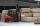To four warehouses is going cement in 25 kg bags. To first one third, to second quarter of the total. The third store got two thirds of the rest, and the last 310 tons came to fourth. How many cement is in all warehouses and how much got every one?
5. Barrel of oilBarrel of oil weighs 283 kg. When it mold 26% oil, weighed 216 kg. What is the mass of the empty barrel?
6. Tea blendTea blends are maked from two kinds of tea. In standard tea mixture are two teas in the ratio 1:3 and 40 g costs 42 CZK. In the premium tea mixture are weighing two teas in the ratio 1:1 and 50 grams costs 60 CZK. How much cost 10 grams of more expensive k
7. Purchase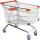Mother bought 5 boxes of milk and 7 kg of potatoes and paid a total CZK 147. Aunt bought 7 boxes of milk and 3 kg of potatoes and paid 131 CZK. What is the price of one carton of milk and 1 kg of potatoes? How CZK together would have saved if bought at the
8. Metal alloy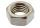What is the ratio of metals in the alloy that is in the 50 tonnes of steel to 30 kg nickel?
9. SpiritFrom 55% and 80% spirit we would like to produce 0.2 kg of 60% spirit. How many of them we must use in a solution?
10. Bob and Bobek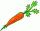Bobek has 2 cabbages and 5 carrots, Bob has 2 cabbages and 9 carrots. When Bobek stepped on the scale weight 5800 g and when Bob weight 8960 g. Actual Bob weight is 8 kg and Bobek 5 kg. How much weighs one carrot?
11. Barrel with liquid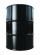Barrel with grain weight 297 kg. When it shed 48% of grain, ha weight 174 kg. What is the weight of empty barrel?
12. HomeworkMother and Kate together weigh 24.5 kg more than the father. Father weighs 7.6 kg more than mother. Father weight 44.8 kg more than Kate. Determine weights of father mother and Kate.
13. Container with water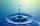The weight of a container with water is 2.48 kg. If the cast 75% water, the container with water has a weight of 0.98 kg. Determine the weight of the empty container. How much water was originally in the container?
14. Coffee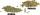Coffee merchant has coffee robusta and arabica species. 1 kg Robusta worth 450 CZK, Arabica 1 kg is 300 CZK more expensive. Calculate how many kilograms of Robusta and Arabica will need to produce 30 kg of the mixture so that the mixture cost is 490 CZK p
15. Children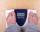Margaret and Zdena weighs the same and Petra 3 kg more. Together weigh 156 kg. How much does they weigh?
16. RingThe ring made from gold and copper alloy has a weight of 14.5 g and a volume of 1.03 cm3. How much gold and how much copper it contains? The metal densities are Au 19.3 g/cm³ and Cu 8.94 kg·dm-3
17. Freight train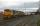The train carries 525 tons of limestone in 29 wagons. Wagons are 15 tonne and 20 tons. How many is 15 ton and how many is 20 ton wagons?